Latest Banking jobs   »

# Quantitative Aptitude Quiz For RBI Grade B Phase 1 2023 -23rd May

Directions (1-5): The following questions are accompanied by two statements A and B. You have to determine which statements(s) is/are sufficient/necessary to answer the questions.
(a) Statement A alone is sufficient to answer the question, but statement B alone is not sufficient to answer the questions.
(b) Statement B alone is sufficient to answer the question, but statement A alone is not sufficient to answer the question.
(c) Both the statements taken together are necessary to answer the questions, but neither of the statements alone is sufficient to answer the question.
(d) Either statement A or statement B by itself is sufficient to answer the question.
(e) Statements A and B taken together are not sufficient to answer the question.

Q1.Calculate SP of article A, If shopkeeper earns a profit of 20% on its CP.
(A) He marked up the price Rs. 75 more than its CP.
(B) Had he given discount of 10% on its MP, he would earn Rs. 7.5 less rupees than actual.

Q2.Find the speed of boat
(A) time taken to cover a distance of 18 km, first in upstream, then in downstream is 4 ½ hours.
(B) If the speed of current gets doubled, then time taken to cover 18 km, first in downstream, then in upstream is 7 ⅕ hours.

Q3.Find the volume of right angle cone?
(A) Curved surface area of cone 18π units and T.S.A. of cone 50% more than curved surface area.
(B) Volume of cylinder of twice the radius as that of cone & √3 time the height as that cone is 324π cubic units.
Q4. Rana told that, his son after x years will be ⅕th of his age, Rana’s wife Negi told that their only son after x years will be ¼th of her age. What is sum of age of Rana and his wife after x years.
(A) The age difference between husband and wife is 9 years.
(B) Value of x is 3.

Q5. A group of people have to collect Rs. 800 for a party and each one of them have to contribute equally. How many peoples were in the group.
(A) Each one of them paid a multiple of 10 and number of people in group was also multiple of 10 and total number of people were less than money paid by each of them.
(B) If 4 people left the group, then each one have to pay an additional Rs. 10

Directions (6-10): In each of the following questions, three statements are given, you have to determine that which statement/statements is/are necessary to answer the question.

Q6. A sum of money Rs 2550 is to be distributed among A, B and C. What will be the share of B?
A. A’s share is 1.5 times B’s share,
B. C’s share is half the share of A and B together.
C. The share of A is Rs 340 more than B.
(a) Either A and B together or A and C together are sufficient
(b) Only A and B together are sufficient
(c) Only A and C together are sufficient
(d) All statements are required
(e) Any 2 of the 3 statements are sufficient

Q7. 40 students sit in rows and columns. How many students are seated in each column?
A. The number of rows is 62.50% of the number of columns.
B. The number of rows is 5/8 of the number of columns.
C. The number of rows is less than the number of columns.
(a) Only A
(b) C and either A or B
(c) Only B
(d) Either A or B
(e) All the statements are required

Q8. A boat takes 2 hours to travel from point A to B in still water. To find out the upstream speed, which of the following information is/are required?
A. Distance between point A and B.
B. Time taken to travel downstream from B to A.
C. Speed of the stream of water.
(a) All are required
(b) Any one pair of A and B, B and C or C and A is sufficient.
(c) Only A and B
(d) Only A and C
(e) None of these

Q9. What is the price of a chair?
A. The price of three tables is equal to the price of seven chairs.
B. The difference between the price of a table and that of a chair is Rs 900.
C. The price of one table is 133 ⅓%  more than the price of one chair.
(a) Only A and B together are sufficient
(b) Only A and C together are sufficient
(c) All together are necessary
(d) B and either A or C together are sufficient
(e) Even all A, B and C together are not sufficient

Q10. A bag contains balls of only three different colors i.e.; red, yellow and green. 3 balls are drawn randomly. What is the probability that the balls drawn are of three different colors?
A. The no. of yellow balls is two more than the no. of red balls.
B. The Sum of the no. of yellow and green balls is three times the no. of red balls.
C. The ratio of the no. of red balls to that of green balls is 3 : 4.
(a) A and either B or C
(b) Any two of them
(c) Only A and C together
(d) Question can’t be answered even after using all the information
(e) All statements are required

Solutions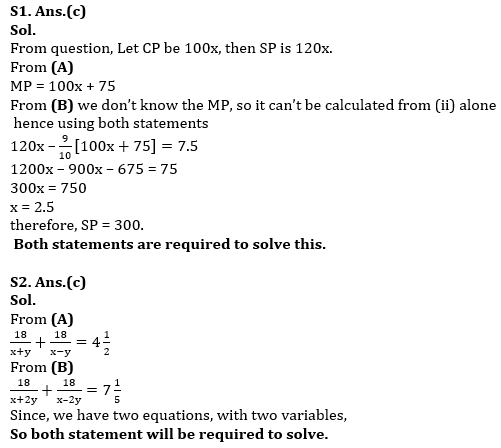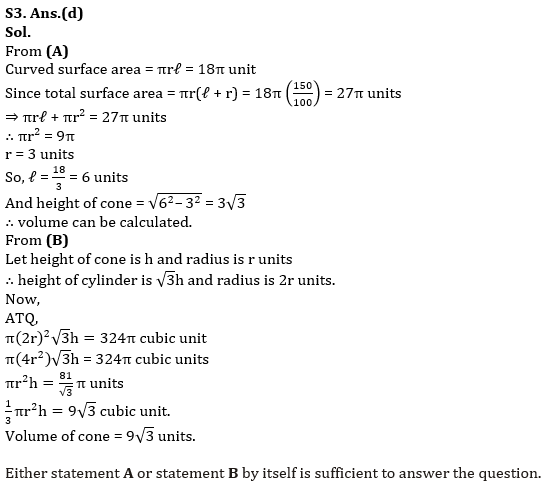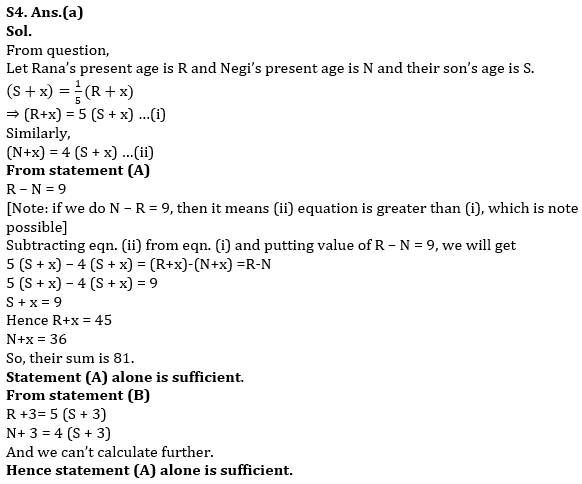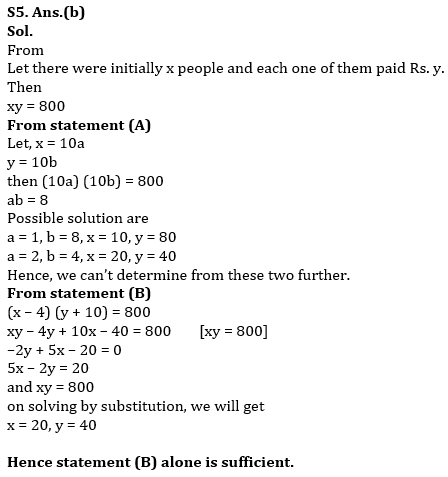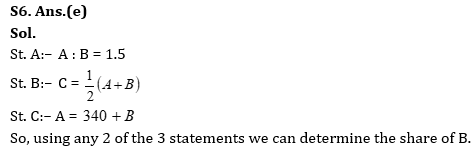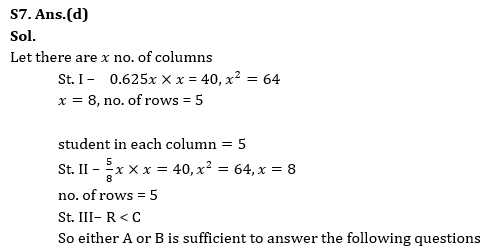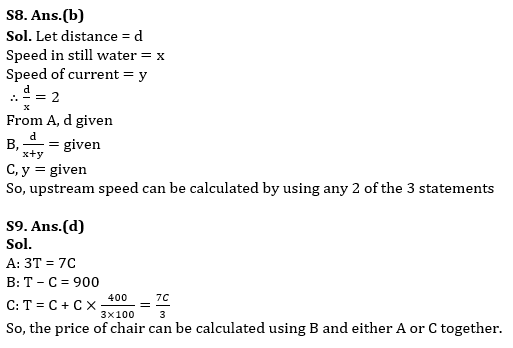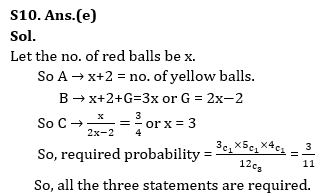## FAQs

### How many sections are there in the RBI Grade B Phase 1 Exam?

There are 4 sections in the RBI Grade B Phase 1 Exam i.e. English Language, General Awareness, Quantitative Aptitude & Reasoning.

#### Congratulations!Union Budget 2023-24: Free PDF